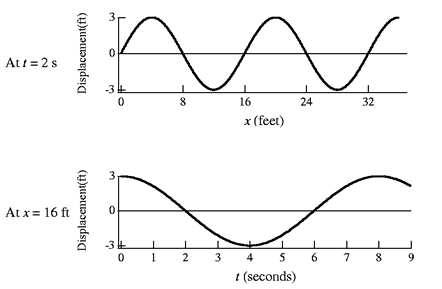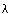TUTORIAL QUESTIONS 85-110

Questions:
85:       Sinusoidal waves in media.
90:       Wave velocity.
95-100:Wave properties from position and time plots.

110:     Relation among frequency, velocity, and wavelength.

Question 85.  A traveling sinusoidal wave can be created in a given medium

a) of any frequency
b) of any wavelength
c) of any wave velocity
d) both a and b
e) a, b, and c

Fundamentals of Sound reference: Secs. 1-G, 1-H.

Question 90.  If you want to make a wave in a string move very fast (i.e., have a large wave velocity), you can

a)  make the tension in the string large
b)  use a thin rather than thick string
c)  jiggle your hand fast to create the wave
d) a and b
e) a, b and c

Fundamentals of Sound reference: Secs. 1-G, 1-H.

Figure for Questions 95-100:Question 95.  What is the wavelength of the sinusoidal wave in the above figure?

Fundamentals of Sound reference: Secs. 1-D, 1-G.

Question 96.  What is the period of the sinusoidal wave in the above figure?

Fundamentals of Sound reference: Secs. 1-D, 1-G.

Question 97.  What is the amplitude of the sinusoidal wave in the above figure?

Fundamentals of Sound reference: Secs. 1-D, 1-G.

Question 98.  What is the wave velocity (or propagation velocity) of the sinusoidal wave in the above figure?

Fundamentals of Sound reference: Secs. 1-C, 1-D, 1-G.

Question 99.  What is the displacement (height) of the string at x = 18 ft and t = 2 s in the above figure?

Fundamentals of Sound reference: Secs. 1-D, 1-G.

Question 100.  What is the displacement (height) of the string at x = 12 ft and t = 6 s in the above figure?

Fundamentals of Sound reference: Secs. 1-D, 1-G.

110.  For traveling sinusoidal waves on strings, water, air, etc.

a) you can have anyand any f so long as the relationship, v= f, holds.
b) only certain wavelengths and frequencies are allowed
c) the wave velocity is totally determined by the medium
d) the wave velocity depends on the dynamic and inertial parameters
e) a and c only
f) b, c and d only
g) a, c and d only
h) none of the above

Fundamentals of Sound reference: Secs. 1-G, 1-H.